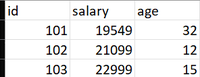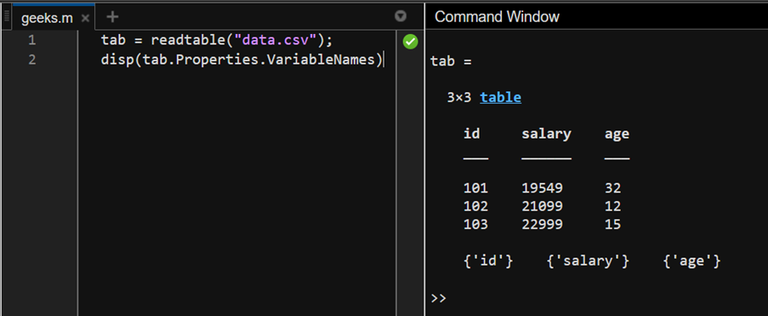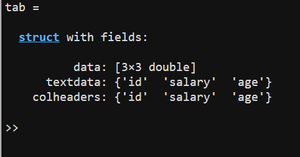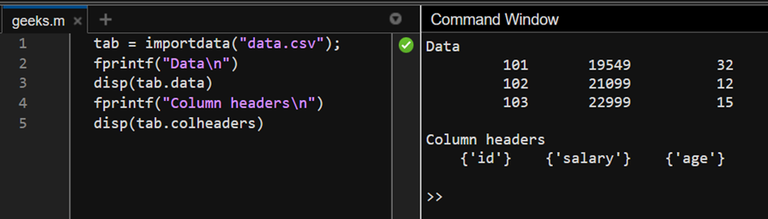Open in App
Not now

# How To Import Data from .CSV File With Numeric Values and Texts Into MATLAB Workspace?

• Last Updated : 22 Mar, 2023

A .csv file is a comma-separated file, in which consecutive data columns are separated by commas and the end of a line is the default EOL character. While dealing with small data, it is common to use a .csv file for storing it. In this article, we shall discuss how to import .csv files, with numeric data and their text headers as the column variables, into a MATLAB workspace.

We can use the simple readable command to import the data from a .csv file as a table with column variables (headers). The readable command default detects the file type and makes the first row the column variables if it is in text form.

### Syntax

See the following example to understand the same.

Example 1:

## Matlab

 `%MATLAB Code``tab = readtable(``"data.csv"``);``disp(tab.Properties.VariableNames)`

In this example, we import a .csv file with numeric data and display its variable names (headers). The following .csv file is used.Now, the above code will give us the following output:Further, this data can be manipulated as per the user’s requirements.

## Import data in MATLAB

There is another way to the importing a .csv file with numeric data and text headers. The import data function of MATLAB allows performing the same functionality as the readable function however, the former creates a struct while the latter creates a table.

### Syntax

tab = importdata(filename)

See the following example to understand the same.

Example 2:

## Matlab

 `%MATLAB Code``tab = importdata(``"data.csv"``);` `%displaying data``fprintf(``"Data\n"``)``disp(tab.data)` `%displaying column headers``fprintf(``"Column headers\n"``)``disp(tab.colheaders)`

This code will import the data as a struct with three fields:

• data
• textdata